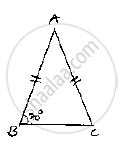Share

Books Shortlist

# In a δAbc, If Ab = Ac and ∠B = 70°, Find ∠A. - CBSE Class 9 - Mathematics

ConceptCongruence of Triangles

#### Question

In a ΔABC, if AB = AC and ∠B = 70°, find ∠A.

#### Solution

Consider , ΔABC we have ∠B=70^@ and AB =AC
Since, AB A C  ABC is an isosceles triangle
⇒ ∠B=∠C      [Angles opposite to equal sides are equal]

⇒∠B = ∠C = 70^@

And also,
Sum of angles in a triangle 180^@

⇒ ∠A+∠B+∠C=180^@

⇒∠A+70^@+70^@=180^@

⇒∠A+140^@=180^@

⇒∠A=180^@-140^@⇒∠A=40^@Is there an error in this question or solution?

#### APPEARS IN

Solution In a δAbc, If Ab = Ac and ∠B = 70°, Find ∠A. Concept: Congruence of Triangles.
S